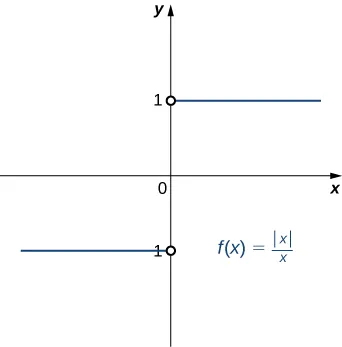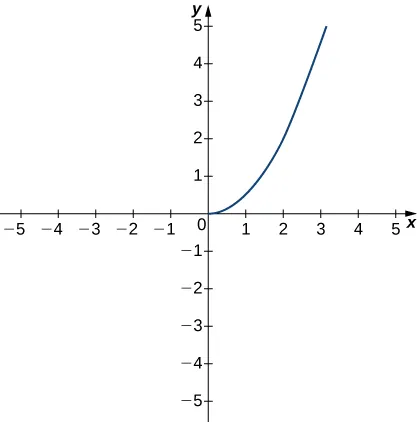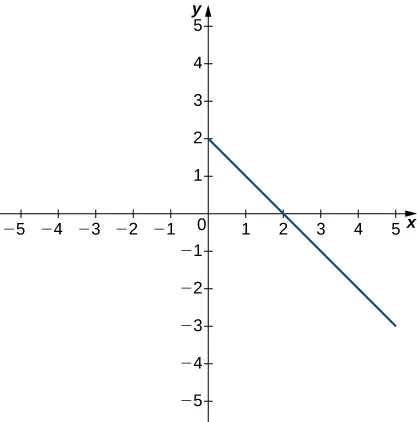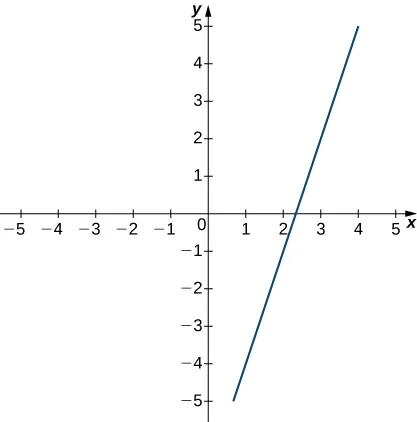Calculus Volume 1

# 2.5The Precise Definition of a Limit

Calculus Volume 12.5 The Precise Definition of a Limit

### Learning Objectives

• 2.5.1 Describe the epsilon-delta definition of a limit.
• 2.5.2 Apply the epsilon-delta definition to find the limit of a function.
• 2.5.3 Describe the epsilon-delta definitions of one-sided limits and infinite limits.
• 2.5.4 Use the epsilon-delta definition to prove the limit laws.

By now you have progressed from the very informal definition of a limit in the introduction of this chapter to the intuitive understanding of a limit. At this point, you should have a very strong intuitive sense of what the limit of a function means and how you can find it. In this section, we convert this intuitive idea of a limit into a formal definition using precise mathematical language. The formal definition of a limit is quite possibly one of the most challenging definitions you will encounter early in your study of calculus; however, it is well worth any effort you make to reconcile it with your intuitive notion of a limit. Understanding this definition is the key that opens the door to a better understanding of calculus.

### Quantifying Closeness

Before stating the formal definition of a limit, we must introduce a few preliminary ideas. Recall that the distance between two points a and b on a number line is given by $|a−b|.|a−b|.$

• The statement $|f(x)−L|<ε|f(x)−L|<ε$ may be interpreted as: The distance between $f(x)f(x)$ and L is less than ε.
• The statement $0<|x−a|<δ0<|x−a|<δ$ may be interpreted as: $x≠ax≠a$ and the distance between x and a is less than δ.

It is also important to look at the following equivalences for absolute value:

• The statement $|f(x)−L|<ε|f(x)−L|<ε$ is equivalent to the statement $L−ε
• The statement $0<|x−a|<δ0<|x−a|<δ$ is equivalent to the statement $a−δ and $x≠a.x≠a.$

With these clarifications, we can state the formal epsilon-delta definition of the limit.

### Definition

Let $f(x)f(x)$ be defined for all $x≠ax≠a$ over an open interval containing a. Let L be a real number. Then

$limx→af(x)=Llimx→af(x)=L$

if, for every $ε>0,ε>0,$ there exists a $δ>0,δ>0,$ such that if $0<|x−a|<δ,0<|x−a|<δ,$ then $|f(x)−L|<ε.|f(x)−L|<ε.$

This definition may seem rather complex from a mathematical point of view, but it becomes easier to understand if we break it down phrase by phrase. The statement itself involves something called a universal quantifier (for every $ε>0),ε>0),$ an existential quantifier (there exists a $δ>0),δ>0),$ and, last, a conditional statement (if $0<|x−a|<δ,0<|x−a|<δ,$ then $|f(x)−L|<ε).|f(x)−L|<ε).$ Let’s take a look at Table 2.9, which breaks down the definition and translates each part.

Definition Translation
1. For every $ε>0,ε>0,$ 1. For every positive distance ε from L,
2. there exists a $δ>0,δ>0,$ 2. There is a positive distance $δδ$ from a,
3. such that 3. such that
4. if $0<|x−a|<δ,0<|x−a|<δ,$ then $|f(x)−L|<ε.|f(x)−L|<ε.$ 4. if x is closer than $δδ$ to a and $x≠a,x≠a,$ then $f(x)f(x)$ is closer than ε to L.
Table 2.9 Translation of the Epsilon-Delta Definition of the Limit

We can get a better handle on this definition by looking at the definition geometrically. Figure 2.39 shows possible values of $δδ$ for various choices of $ε>0ε>0$ for a given function $f(x),f(x),$ a number a, and a limit L at a. Notice that as we choose smaller values of ε (the distance between the function and the limit), we can always find a $δδ$ small enough so that if we have chosen an x value within $δδ$ of a, then the value of $f(x)f(x)$ is within ε of the limit L.

Figure 2.39 These graphs show possible values of $δδ$, given successively smaller choices of ε.

### Media

Visit the following applet to experiment with finding values of $δδ$ for selected values of ε:

Example 2.39 shows how you can use this definition to prove a statement about the limit of a specific function at a specified value.

### Example 2.39

#### Proving a Statement about the Limit of a Specific Function

Prove that $limx→1(2x+1)=3.limx→1(2x+1)=3.$

#### Analysis

In this part of the proof, we started with $|(2x+1)−3||(2x+1)−3|$ and used our assumption $0<|x−1|<δ0<|x−1|<δ$ in a key part of the chain of inequalities to get $|(2x+1)−3||(2x+1)−3|$ to be less than ε. We could just as easily have manipulated the assumed inequality $0<|x−1|<δ0<|x−1|<δ$ to arrive at $|(2x+1)−3|<ε|(2x+1)−3|<ε$ as follows:

$0<|x−1|<δ⇒|x−1|<δ⇒−δ

Therefore, $limx→1(2x+1)=3.limx→1(2x+1)=3.$ (Having completed the proof, we state what we have accomplished.)

After removing all the remarks, here is a final version of the proof:

Let $ε>0.ε>0.$

Choose $δ=ε/2.δ=ε/2.$

Assume $0<|x−1|<δ.0<|x−1|<δ.$

Thus,

$|(2x+1)−3|=|2x−2|=|2(x−1)|=|2||x−1|=2|x−1|<2·δ=2·ε2=ε.|(2x+1)−3|=|2x−2|=|2(x−1)|=|2||x−1|=2|x−1|<2·δ=2·ε2=ε.$

Therefore, $limx→1(2x+1)=3.limx→1(2x+1)=3.$

The following Problem-Solving Strategy summarizes the type of proof we worked out in Example 2.39.

### Problem-Solving Strategy

#### Problem-Solving Strategy: Proving That $limx→af(x)=Llimx→af(x)=L$ for a Specific Function $f(x)f(x)$

1. Let’s begin the proof with the following statement: Let $ε>0.ε>0.$
2. Next, we need to obtain a value for $δ.δ.$ After we have obtained this value, we make the following statement, filling in the blank with our choice of $δδ$: Choose $δ=_______.δ=_______.$
3. The next statement in the proof should be (at this point, we fill in our given value for a):
Assume $0<|x−a|<δ.0<|x−a|<δ.$
4. Next, based on this assumption, we need to show that $|f(x)−L|<ε,|f(x)−L|<ε,$ where $f(x)f(x)$ and L are our function $f(x)f(x)$ and our limit L. At some point, we need to use $0<|x−a|<δ.0<|x−a|<δ.$
5. We conclude our proof with the statement: Therefore, $limx→af(x)=L.limx→af(x)=L.$

### Example 2.40

#### Proving a Statement about a Limit

Complete the proof that $limx→−1(4x+1)=−3limx→−1(4x+1)=−3$ by filling in the blanks.

Let _____.

Choose $δ=_______.δ=_______.$

Assume $0<|x−_______|<δ.0<|x−_______|<δ.$

Thus, $|________−________|=_____________________________________ε.|________−________|=_____________________________________ε.$

### Checkpoint2.27

Complete the proof that $limx→2(3x−2)=4limx→2(3x−2)=4$ by filling in the blanks.

Let _______.

Choose $δ=_______.δ=_______.$

Assume $0<|x−____|<____.0<|x−____|<____.$

Thus,

$|_______−____|=______________________________ε.|_______−____|=______________________________ε.$

Therefore, $limx→2(3x−2)=4.limx→2(3x−2)=4.$

In Example 2.39 and Example 2.40, the proofs were fairly straightforward, since the functions with which we were working were linear. In Example 2.41, we see how to modify the proof to accommodate a nonlinear function.

### Example 2.41

#### Proving a Statement about the Limit of a Specific Function (Geometric Approach)

Prove that $limx→2x2=4.limx→2x2=4.$

### Checkpoint2.28

Find δ corresponding to $ε>0ε>0$ for a proof that $limx→9x=3.limx→9x=3.$

The geometric approach to proving that the limit of a function takes on a specific value works quite well for some functions. Also, the insight into the formal definition of the limit that this method provides is invaluable. However, we may also approach limit proofs from a purely algebraic point of view. In many cases, an algebraic approach may not only provide us with additional insight into the definition, it may prove to be simpler as well. Furthermore, an algebraic approach is the primary tool used in proofs of statements about limits. For Example 2.42, we take on a purely algebraic approach.

### Example 2.42

#### Proving a Statement about the Limit of a Specific Function (Algebraic Approach)

Prove that $limx→−1(x2−2x+3)=6.limx→−1(x2−2x+3)=6.$

### Checkpoint2.29

Complete the proof that $limx→1x2=1.limx→1x2=1.$

Let $ε>0;ε>0;$ choose $δ=min{1,ε/3};δ=min{1,ε/3};$ assume $0<|x−1|<δ.0<|x−1|<δ.$

Since $|x−1|<1,|x−1|<1,$ we may conclude that $−1 Thus, $1 Hence, $|x+1|<3.|x+1|<3.$

You will find that, in general, the more complex a function, the more likely it is that the algebraic approach is the easiest to apply. The algebraic approach is also more useful in proving statements about limits.

### Proving Limit Laws

We now demonstrate how to use the epsilon-delta definition of a limit to construct a rigorous proof of one of the limit laws. The triangle inequality is used at a key point of the proof, so we first review this key property of absolute value.

### Definition

The triangle inequality states that if a and b are any real numbers, then $|a+b|≤|a|+|b|.|a+b|≤|a|+|b|.$

#### Proof

We prove the following limit law: If $limx→af(x)=Llimx→af(x)=L$ and $limx→ag(x)=M,limx→ag(x)=M,$ then $limx→a(f(x)+g(x))=L+M.limx→a(f(x)+g(x))=L+M.$

Let $ε>0.ε>0.$

Choose $δ1>0δ1>0$ so that if $0<|x−a|<δ1,0<|x−a|<δ1,$ then $|f(x)−L|<ε/2.|f(x)−L|<ε/2.$

Choose $δ2>0δ2>0$ so that if $0<|x−a|<δ2,0<|x−a|<δ2,$ then $|g(x)−M|<ε/2.|g(x)−M|<ε/2.$

Choose $δ=min{δ1,δ2}.δ=min{δ1,δ2}.$

Assume $0<|x−a|<δ.0<|x−a|<δ.$

Thus,

$0<|x−a|<δ1and0<|x−a|<δ2.0<|x−a|<δ1and0<|x−a|<δ2.$

Hence,

$|(f(x)+g(x))−(L+M)|=|(f(x)−L)+(g(x)−M)|≤|f(x)−L|+|g(x)−M|<ε2+ε2=ε.|(f(x)+g(x))−(L+M)|=|(f(x)−L)+(g(x)−M)|≤|f(x)−L|+|g(x)−M|<ε2+ε2=ε.$

We now explore what it means for a limit not to exist. The limit $limx→af(x)limx→af(x)$ does not exist if there is no real number L for which $limx→af(x)=L.limx→af(x)=L.$ Thus, for all real numbers L, $limx→af(x)≠L.limx→af(x)≠L.$ To understand what this means, we look at each part of the definition of $limx→af(x)=Llimx→af(x)=L$ together with its opposite. A translation of the definition is given in Table 2.10.

Definition Opposite
1. For every $ε>0,ε>0,$ 1. There exists $ε>0ε>0$ so that
2. there exists a $δ>0,δ>0,$ so that 2. for every $δ>0,δ>0,$
3. if $0<|x−a|<δ,0<|x−a|<δ,$ then $|f(x)−L|<ε.|f(x)−L|<ε.$ 3. There is an x satisfying $0<|x−a|<δ0<|x−a|<δ$ so that $|f(x)−L|≥ε.|f(x)−L|≥ε.$
Table 2.10 Translation of the Definition of $lim x → a f ( x ) = L lim x → a f ( x ) = L$ and its Opposite

Finally, we may state what it means for a limit not to exist. The limit $limx→af(x)limx→af(x)$ does not exist if for every real number L, there exists a real number $ε>0ε>0$ so that for all $δ>0,δ>0,$ there is an x satisfying $0<|x−a|<δ,0<|x−a|<δ,$ so that $|f(x)−L|≥ε.|f(x)−L|≥ε.$ Let’s apply this in Example 2.43 to show that a limit does not exist.

### Example 2.43

#### Showing That a Limit Does Not Exist

Show that $limx→0|x|xlimx→0|x|x$ does not exist. The graph of $f(x)=|x|/xf(x)=|x|/x$ is shown here:### One-Sided and Infinite Limits

Just as we first gained an intuitive understanding of limits and then moved on to a more rigorous definition of a limit, we now revisit one-sided limits. To do this, we modify the epsilon-delta definition of a limit to give formal epsilon-delta definitions for limits from the right and left at a point. These definitions only require slight modifications from the definition of the limit. In the definition of the limit from the right, the inequality $0 replaces $0<|x−a|<δ,0<|x−a|<δ,$ which ensures that we only consider values of x that are greater than (to the right of) a. Similarly, in the definition of the limit from the left, the inequality $−δ replaces $0<|x−a|<δ,0<|x−a|<δ,$ which ensures that we only consider values of x that are less than (to the left of) a.

### Definition

Limit from the Right: Let $f(x)f(x)$ be defined over an open interval of the form $(a,b)(a,b)$ where $a Then,

$limx→a+f(x)=Llimx→a+f(x)=L$

if for every $ε>0,ε>0,$ there exists a $δ>0δ>0$ such that if $0 then $|f(x)−L|<ε.|f(x)−L|<ε.$

Limit from the Left: Let $f(x)f(x)$ be defined over an open interval of the form $(b,c)(b,c)$ where $b Then,

$limx→a−f(x)=Llimx→a−f(x)=L$

if for every $ε>0,ε>0,$ there exists a $δ>0δ>0$ such that if $−δ then $|f(x)−L|<ε.|f(x)−L|<ε.$

### Example 2.44

#### Proving a Statement about a Limit From the Right

Prove that $limx→4+x−4=0.limx→4+x−4=0.$

### Checkpoint2.30

Find $δδ$ corresponding to ε for a proof that $limx→1−1−x=0.limx→1−1−x=0.$

We conclude the process of converting our intuitive ideas of various types of limits to rigorous formal definitions by pursuing a formal definition of infinite limits. To have $limx→af(x)=+∞,limx→af(x)=+∞,$ we want the values of the function $f(x)f(x)$ to get larger and larger as x approaches a. Instead of the requirement that $|f(x)−L|<ε|f(x)−L|<ε$ for arbitrarily small ε when $0<|x−a|<δ0<|x−a|<δ$ for small enough $δ,δ,$ we want $f(x)>Mf(x)>M$ for arbitrarily large positive M when $0<|x−a|<δ0<|x−a|<δ$ for small enough $δ.δ.$ Figure 2.43 illustrates this idea by showing the value of $δδ$ for successively larger values of M.

Figure 2.43 These graphs plot values of $δδ$ for M to show that $limx→af(x)=+∞.limx→af(x)=+∞.$

### Definition

Let $f(x)f(x)$ be defined for all $x≠ax≠a$ in an open interval containing a. Then, we have an infinite limit

$limx→af(x)=+∞limx→af(x)=+∞$

if for every $M>0,M>0,$ there exists $δ>0δ>0$ such that if $0<|x−a|<δ,0<|x−a|<δ,$ then $f(x)>M.f(x)>M.$

Let $f(x)f(x)$ be defined for all $x≠ax≠a$ in an open interval containing a. Then, we have a negative infinite limit

$limx→af(x)=−∞limx→af(x)=−∞$

if for every $M>0,M>0,$ there exists $δ>0δ>0$ such that if $0<|x−a|<δ,0<|x−a|<δ,$ then $f(x)<−M.f(x)<−M.$

### Section 2.5 Exercises

In the following exercises, write the appropriate $εε$-$δδ$ definition for each of the given statements.

176.

$lim x → a f ( x ) = N lim x → a f ( x ) = N$

177.

$lim t → b g ( t ) = M lim t → b g ( t ) = M$

178.

$lim x → c h ( x ) = L lim x → c h ( x ) = L$

179.

$lim x → a φ ( x ) = A lim x → a φ ( x ) = A$

The following graph of the function f satisfies $limx→2f(x)=2.limx→2f(x)=2.$ In the following exercises, determine a value of $δ>0δ>0$ that satisfies each statement.180.

If $0<|x−2|<δ,0<|x−2|<δ,$ then $|f(x)−2|<1.|f(x)−2|<1.$

181.

If $0<|x−2|<δ,0<|x−2|<δ,$ then $|f(x)−2|<0.5.|f(x)−2|<0.5.$

The following graph of the function f satisfies $limx→3f(x)=−1.limx→3f(x)=−1.$ In the following exercises, determine a value of $δ>0δ>0$ that satisfies each statement.182.

If $0<|x−3|<δ,0<|x−3|<δ,$ then $|f(x)+1|<1.|f(x)+1|<1.$

183.

If $0<|x−3|<δ,0<|x−3|<δ,$ then $|f(x)+1|<2.|f(x)+1|<2.$

The following graph of the function f satisfies $limx→3f(x)=2.limx→3f(x)=2.$ In the following exercises, for each value of ε, find a value of $δ>0δ>0$ such that the precise definition of limit holds true.184.

$ε = 1.5 ε = 1.5$

185.

$ε = 3 ε = 3$

[T] In the following exercises, use a graphing calculator to find a number $δδ$ such that the statements hold true.

186.

$|sin(2x)−12|<0.1,|sin(2x)−12|<0.1,$ whenever $|x−π12|<δ|x−π12|<δ$

187.

$| x − 4 − 2 | < 0.1 , whenever | x − 8 | < δ | x − 4 − 2 | < 0.1 , whenever | x − 8 | < δ$

In the following exercises, use the precise definition of limit to prove the given limits.

188.

$lim x → 2 ( 5 x + 8 ) = 18 lim x → 2 ( 5 x + 8 ) = 18$

189.

$lim x → 3 x 2 − 9 x − 3 = 6 lim x → 3 x 2 − 9 x − 3 = 6$

190.

$lim x → 2 2 x 2 − 3 x − 2 x − 2 = 5 lim x → 2 2 x 2 − 3 x − 2 x − 2 = 5$

191.

$lim x → 0 x 4 = 0 lim x → 0 x 4 = 0$

192.

$lim x → 2 ( x 2 + 2 x ) = 8 lim x → 2 ( x 2 + 2 x ) = 8$

In the following exercises, use the precise definition of limit to prove the given one-sided limits.

193.

$lim x → 5 − 5 − x = 0 lim x → 5 − 5 − x = 0$

194.

$lim x → 0 + f ( x ) = −2 , where f ( x ) = { 8 x − 3 , if x < 0 4 x − 2 , if x ≥ 0 . lim x → 0 + f ( x ) = −2 , where f ( x ) = { 8 x − 3 , if x < 0 4 x − 2 , if x ≥ 0 .$

195.

$lim x → 1 − f ( x ) = 3 , where f ( x ) = { 5 x − 2 , if x < 1 7 x − 1 , if x ≥ 1 . lim x → 1 − f ( x ) = 3 , where f ( x ) = { 5 x − 2 , if x < 1 7 x − 1 , if x ≥ 1 .$

In the following exercises, use the precise definition of limit to prove the given infinite limits.

196.

$lim x → 0 1 x 2 = ∞ lim x → 0 1 x 2 = ∞$

197.

$lim x → −1 3 ( x + 1 ) 2 = ∞ lim x → −1 3 ( x + 1 ) 2 = ∞$

198.

$lim x → 2 − 1 ( x − 2 ) 2 = − ∞ lim x → 2 − 1 ( x − 2 ) 2 = − ∞$

199.

An engineer is using a machine to cut a flat square of Aerogel of area 144 cm2. If there is a maximum error tolerance in the area of 8 cm2, how accurately must the engineer cut on the side, assuming all sides have the same length? How do these numbers relate to $δ,δ,$ ε, a, and L?

200.

Use the precise definition of limit to prove that the following limit does not exist: $limx→1|x−1|x−1.limx→1|x−1|x−1.$

201.

Using precise definitions of limits, prove that $limx→0f(x)limx→0f(x)$ does not exist, given that $f(x)f(x)$ is the ceiling function. (Hint: Try any $δ<1.)δ<1.)$

202.

Using precise definitions of limits, prove that $limx→0f(x)limx→0f(x)$ does not exist: $f(x)={1ifxis rational0ifxis irrational.f(x)={1ifxis rational0ifxis irrational.$ (Hint: Think about how you can always choose a rational number $0 but $|f(r)−0|=1.)|f(r)−0|=1.)$

203.

Using precise definitions of limits, determine $limx→0f(x)limx→0f(x)$ for $f(x)={xifxis rational0ifxis irrational.f(x)={xifxis rational0ifxis irrational.$ (Hint: Break into two cases, x rational and x irrational.)

204.

Using the function from the previous exercise, use the precise definition of limits to show that $limx→af(x)limx→af(x)$ does not exist for $a≠0.a≠0.$

For the following exercises, suppose that $limx→af(x)=Llimx→af(x)=L$ and $limx→ag(x)=Mlimx→ag(x)=M$ both exist. Use the precise definition of limits to prove the following limit laws:

205.

$lim x → a ( f ( x ) + g ( x ) ) = L + M lim x → a ( f ( x ) + g ( x ) ) = L + M$

206.

$limx→a[cf(x)]=cLlimx→a[cf(x)]=cL$ for any real constant c (Hint: Consider two cases: $c=0c=0$ and $c≠0.)c≠0.)$

207.

$limx→a[f(x)g(x)]=LM.limx→a[f(x)g(x)]=LM.$ (Hint: $|f(x)g(x)−LM|=|f(x)g(x)−LM|=$ $|f(x)g(x)−f(x)M+f(x)M−LM|≤|f(x)||g(x)−M|+|M||f(x)−L|.)|f(x)g(x)−f(x)M+f(x)M−LM|≤|f(x)||g(x)−M|+|M||f(x)−L|.)$

Order a print copy

As an Amazon Associate we earn from qualifying purchases.